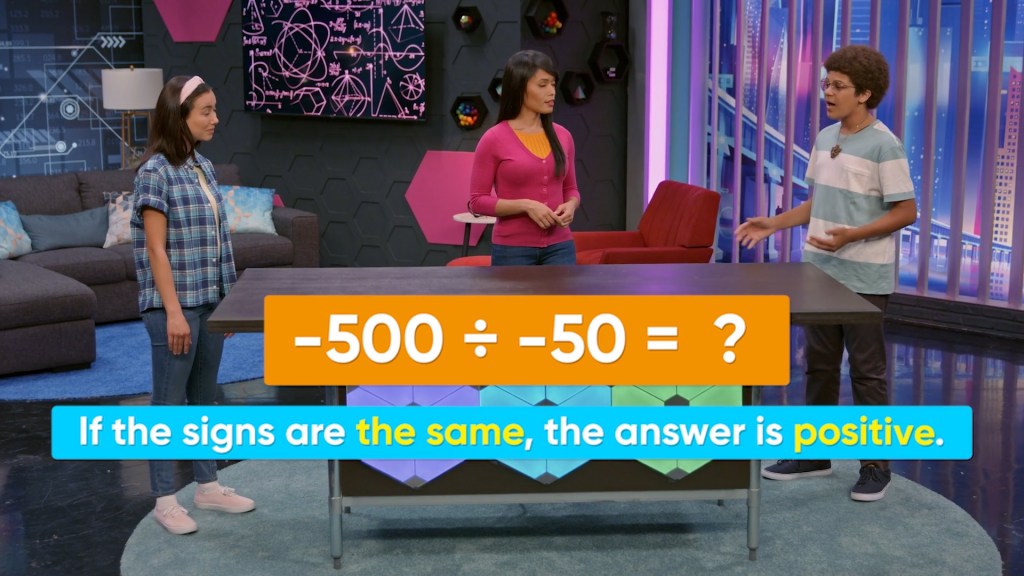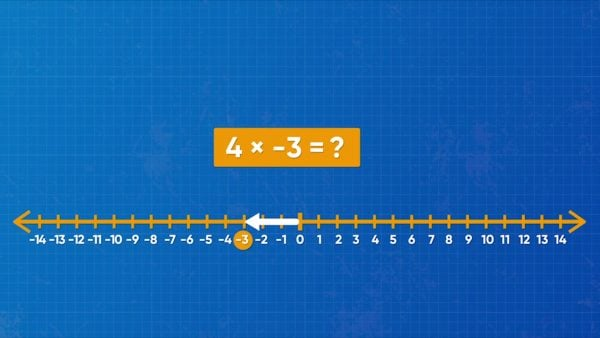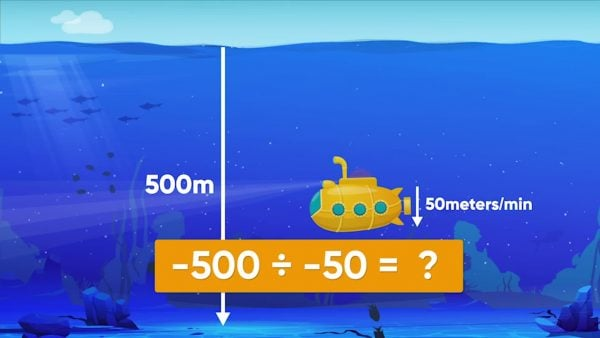Multiply & Divide with Negative Numbers Video | 6th, 7th & 8th Grade
1%
It was processed successfully!WHAT IS MULTIPLYING & DIVIDING WITH NEGATIVE NUMBERS?

You already know how to multiply and divide positive numbers. When one or more of the numbers are negative, you can find the product or quotient in the same way. The only difference is that you need to decide the sign of the result.

To better understand Multiplying & Dividing with Negative Numbers…

WHAT IS MULTIPLYING & DIVIDING WITH NEGATIVE NUMBERS?. You already know how to multiply and divide positive numbers. When one or more of the numbers are negative, you can find the product or quotient in the same way. The only difference is that you need to decide the sign of the result. To better understand Multiplying & Dividing with Negative Numbers…

## LET’S BREAK IT DOWN!

### Multiply a positive number by a negative number.A weather report says that the temperature will decrease by 3 degrees each day for 4 days. Represent the daily decrease of 3 degrees as the integer −3. Since the temperature changes −3 degrees for 4 days you can represent the total temperature change (decrease) as (-3) + (-3) + (-3) + (-3) or 4 × (-3). You can use a number line to help you find the product. Start at 0. Move 3 places left a total of 4 times. You land on -12. This means that 4 × (-3) = -12. When you multiply and exactly one factor is negative, the result is negative. This is because you are repeatedly adding a negative number, so the answer must be negative! Try this yourself: What is (−10) × 8?

Multiply a positive number by a negative number. A weather report says that the temperature will decrease by 3 degrees each day for 4 days. Represent the daily decrease of 3 degrees as the integer −3. Since the temperature changes −3 degrees for 4 days you can represent the total temperature change (decrease) as (-3) + (-3) + (-3) + (-3) or 4 × (-3). You can use a number line to help you find the product. Start at 0. Move 3 places left a total of 4 times. You land on -12. This means that 4 × (-3) = -12. When you multiply and exactly one factor is negative, the result is negative. This is because you are repeatedly adding a negative number, so the answer must be negative! Try this yourself: What is (−10) × 8?

### Multiply a negative number by a negative number.Learning how to multiply two negative numbers can help you understand the stock market. People who analyze the stock market have to ‘take away losses.’ This means that they multiply a negative number by a negative number. You know that (-4) × 5 = -20. On the number line, this value is 20 places to the left of 0. When we make something negative, it is the same as changing its direction on the number line. When you introduce another negative to the expression by multiplying (-4) × (−5), change the direction on the number line. So, the product is 20, which is positive. When you multiply two negative numbers, the product is positive. Try this yourself: Is the product (-55) × (-5) positive or negative?

Multiply a negative number by a negative number. Learning how to multiply two negative numbers can help you understand the stock market. People who analyze the stock market have to ‘take away losses.’ This means that they multiply a negative number by a negative number. You know that (-4) × 5 = -20. On the number line, this value is 20 places to the left of 0. When we make something negative, it is the same as changing its direction on the number line. When you introduce another negative to the expression by multiplying (-4) × (−5), change the direction on the number line. So, the product is 20, which is positive. When you multiply two negative numbers, the product is positive. Try this yourself: Is the product (-55) × (-5) positive or negative?

### Divide a negative number by a positive number.You and two of your friends borrow \$240 to start a skateboard chair company. The total owing is \$240, which can be represented as -\$240. How much do each of you owe? -240 ÷ 3 = ? This is the same as ? × 3 = -240. For the product to be negative, the missing factor must be negative. -240 ÷ 3 = −80. Each person owes \$80, represented by -\$80. A negative number divided by a positive number is always a negative number. What if the number being divided is negative? 240 ÷(-3) = ? is the same as ? × (−3) = 240. For the product to be positive, the missing factor must be negative. So, 240 ÷(-3) = −80. Try this yourself: What is (-66) ÷6?

Divide a negative number by a positive number. You and two of your friends borrow \$240 to start a skateboard chair company. The total owing is \$240, which can be represented as -\$240. How much do each of you owe? -240 ÷ 3 = ? This is the same as ? × 3 = -240. For the product to be negative, the missing factor must be negative. -240 ÷ 3 = −80. Each person owes \$80, represented by -\$80. A negative number divided by a positive number is always a negative number. What if the number being divided is negative? 240 ÷(-3) = ? is the same as ? × (−3) = 240. For the product to be positive, the missing factor must be negative. So, 240 ÷(-3) = −80. Try this yourself: What is (-66) ÷6?

### Divide a negative number by a negative number.If you want to land a drone 500 meters below sea level, you can represent this distance as −500. If you descend at 50 meters per minute, you can represent this number as −50. To find out how long it takes to get to 500 meters below sea level, divide −500 by −50. −500 -50= ? This is the same as ? × (−5) = −500. For the product to be negative, the missing factor must be positive! −500 -50= 10. It takes 10 minutes to descend to 500 meters below sea level at 50 meters per minute. Try this yourself: What is (−100) ÷ (−5)?

Divide a negative number by a negative number. If you want to land a drone 500 meters below sea level, you can represent this distance as −500. If you descend at 50 meters per minute, you can represent this number as −50. To find out how long it takes to get to 500 meters below sea level, divide −500 by −50. −500 -50= ? This is the same as ? × (−5) = −500. For the product to be negative, the missing factor must be positive! −500 -50= 10. It takes 10 minutes to descend to 500 meters below sea level at 50 meters per minute. Try this yourself: What is (−100) ÷ (−5)?

## MULTIPLY & DIVIDE WITH NEGATIVE NUMBERS VOCABULARY

Integer
The set of whole numbers and negative whole numbers.
Whole Number
The set of counting numbers and zero.
Absolute Value
The distance of a number from 0 on the number line.
Factor
A number being multiplied in a multiplication problem.
Product
The result of multiplying.
Dividend
The number being divided in a division problem.
The dividing number in a division problem.
The result of dividing.
A number to the right of zero on the number line.
A number to the left of zero on the number line.
A number that is the same distance from zero, but on the other side of zero on the number line.

## MULTIPLY & DIVIDE WITH NEGATIVE NUMBERS DISCUSSION QUESTIONS

### Two integers are multiplied, and their product is negative. What must be true about the numbers?

One number must be positive and the other must be negative.

### Two integers are divided, and their quotient is positive. What must be true about the numbers?

The numbers (dividend and divisor) both have the same sign.

### How are the rules for multiplying and dividing integers related?

The sign of the product and of the quotient follow the same rules in relation to the sign of the two numbers being multiplied or divided. If the numbers have the same sign, the product or quotient is positive. If they have different signs, the product or quotient is negative.

### Is the product (−5) × (−2) × (−3) positive or negative? Explain.

The product is negative since there is an odd number of negative factors. Each pair of negative factors results in a positive product, which is then multiplied by a negative number.